FUZZEKS [to Index]
Fuzzy Kriging: Basics / Theoretical concepts

Kriging belongs to the most popular methods of spatial interpolation of data. The fuzzy kriging abilities of FUZZEKS are based on a modification of the conventional kriging procedure. The difference is that fuzzy kriging (of the type FUZZEKS uses) additionally allows the use of imprecise input data (e.g. estimations obtained from an expert).

Imprecise data are represented in FUZZEKS by fuzzy numbers.

Fuzzy numbers (definition)

The definition of a fuzzy number is based on the definition of a fuzzy set.

A fuzzy set of a set X is defined by a membership function m that supplies for each value of X a so-called membership value in the range [0,1].
m : X -> [0,1]
It is similar to a subset of X, except each element x of X can not only be (m(x)=1) or not be (m(x)=0) element of the fuzzy set, but also can be element gradually (0<m(x)<1).

A fuzzy set of R (the real numbers) is called a fuzzy number if the membership function m is convex and m(x)=1 for exactly one point x of R.
(Convex means that the function decreases monotonically to both sides of the point where the value 1 is taken.)

Have a look at "Load Input File / FDF-inputfile-format" for further details on how to formulate fuzzy input data for FUZZEKS.

Example: Definition of a fuzzy number 4. If values of the parameter x can not be lower than 2 and not greater than 5 then a membership function may look like: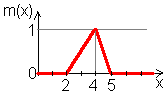(In this example: m(2)=0, m(3)=0.5, m(4)=1, etc.)
For FUZZEKS one could define this fuzzy number in the form
4 / 0: 2-5
(first the point where the membership function takes the value 1, then the range of values with membership values grater than 0).

Remark: The conventional crisp real numbers can be embedded in the set of fuzzy numbers, so that crisp numbers are a special case of fuzzy numbers. Their membership function is 1 for the given real number and 0 for all other real numbers. This is because a crisp real number always expresses only this number (all other numbers are impossible, so the membership value is 0).

Fuzzy kriging procedure

The input for fuzzy kriging are values of parameters (either exact or imprecise) at given points (the parameters that are to be estimated).
The output of fuzzy kriging is an estimated value for any specified location.

Fuzzy kriging is based on a theoretical variogram which is result of a statistical analysis of the parameter. It tells about the similarity of values with respect to their distance.

The experimental variogram (calculated utilizing the input data) is used as a statistical tool to find the theoretical variogram. FUZZEKS can calculate it taking the fuzziness into account (which yields a fuzzy experimental variogram), although the theoretical variogram can only be crisp. The reason is that the user can take the fuzziness into account when fitting a theoretical variogram curve.

Remark: The experimental (semi)variogram can be formulated as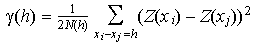where the xi are the input coordinates, the Z(xi) are the input values which can take a form of fuzzy numbers mentioned above, and h is the distance-vector between points. In practice the direction of the distance-vector is not taken into account (in this case the vector xi-xj is replaced by the distance of xi and xj).

To facilitate the kriging procedure FUZZEKS offers

Theoretical concepts of fuzzy kriging

The rest of this page deals with the (mathematical) assumptions that are made for simple point kriging. Also, it roughly describes how the assumptions lead to the spatial kriging estimations.

The following description is valid for the crisp case. If fuzzy values are used, the Extension Principle (well known in fuzzy theory, see also references at bottom of Overview/References, especially [Zadeh, 1965]) is used to convert the main kriging equation (3 headlines below) into a fuzzy function.
(If you want to have a rough description of the application of the extension principle, have a look at the Overview of membership function window.) Below (at the main kriging equation) is an additional remark regarding the fuzzy version of the main kriging equation.

Notations

x: Location in 2-dimensional space
Z(x): Input parameter value at the location x
Z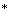(x): Estimated value at the location x
xi: Locations where an input parameter value exists (i=1..n)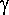(x1,x2)=(x1-x2): The semivariogram (=VAR(Z(x1)-Z(x2))/2), which is the same as the earlier definition of the experimental variogram because of the following assumptions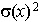: Kriging variance at the location x ( E((Z(x)-Z(x))^2) )

Assumptions

(1) E(Z(x))=m
The expected parameter value exists and does not depend on x.

(2) VAR(Z(x+h)-Z(x))/2=(h)
The difference Z(x+h)-Z(x) has finite variance
and does not depend on x.

Main kriging equation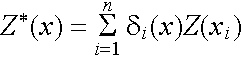The estimated value is a linear combination of the input values and the coefficients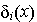. Theare calculated by using the assumptions with a user-supplied theoretical (semi)variogram.
(Remark: For a fixed x the coefficientsare variables of real numbers only.)

Remark for the case of fuzzy input values Z(x): Theare crisp values although the input values are fuzzy (in the case of a crisp theoretical variogram, which is true for FUZZEKS as described above); they can be obtained by the crisp solution (which is described below).

Calculation of theThe assumptions and the demand
E(Z(x)-Z(x)) = 0
(1)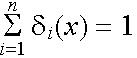(2)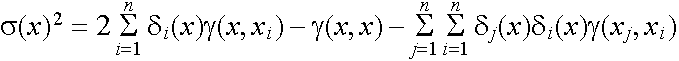Theare chosen in a way that a minimal estimation variance (2) will be obtained (observing (1)).